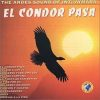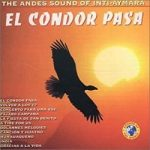##Simon and Garfunkel – El condor pasa## El condor pasa

Single de: Simon and Garfunkel
Extrait de l'albumBridge Over Troubled Water
Sortie: 1970
Genre: Folklorike
Auteur-compositeurSimon and GarfunkelNiveau Expert

CAPO II

Am(STOP)

I'd rather be a sparrow than a snail
Yes,    I would
If     I   could
I surely would

Am(x)   G(x)

Am   Am(x)   G(x)

Away, I'd rather sail away
Like a swan    that's here and gone
A man gets tied up to the ground
He gives the world        its saddest sound

Am(x)   G(x)

Am   Am(x)   G(x)

Am  Am

I'd rather be a hammer than a nail

Yes,   I would
If  I only     could
I surely would

Am(x)   G(x)

Am   Am(x)   G(x)

Away, I'd rather sail away
Like a swan    that's here and gone
A man gets tied up to the ground
He gives the world        its saddest sound

Am(x)   G(x)

Am   Am(x)   G(x)

Am  Am

I'd rather be a forest than a street

Yes,    I would
If     I   could
I surely would

Am(x)   G(x)

Am   Am(x)   G(x)

Away, I'd rather sail away
Like a swan    that's here and gone
A man gets tied up to the ground
He gives the world        its saddest sound

Am(x)   G(x)

Am   Am(x)   G(x)

Am  Am

I'd rather feel the earth beneath my feet

Yes,   I would
If  I only     could
I surely would

Am(x)   G(x)

Am   Am(x)   G(x)

Away, I'd rather sail away
Like a swan    that's here and gone
A man gets tied up to the ground
He gives the world        its saddest sound

Am(x)   G(x)

Am   Am(x)   G(x)

Am(STOP)

CAPO II

Em(STOP)

I'd rather be a sparrow than a snail
Yes,    I would
If     I   could
I surely would

Em(x)   D(x)

Em    Em(x)   D(x)

Away, I'd rather sail away
Like a swan    that's here and gone
A man gets tied up to the ground
He gives the world        its saddest sound

Em(x)   D(x)

Em    Em(x)   D(x)

Em   Em

I'd rather be a hammer than a nail

Yes,   I would
If  I only     could
I surely would

Em(x)   D(x)

Em    Em(x)   D(x)

Away, I'd rather sail away
Like a swan    that's here and gone
A man gets tied up to the ground
He gives the world        its saddest sound

Em(x)   D(x)

Em    Em(x)   D(x)

Em   Em

I'd rather be a forest than a street

Yes,    I would
If     I   could
I surely would

Em(x)   D(x)

Em    Em(x)   D(x)

Away, I'd rather sail away
Like a swan    that's here and gone
A man gets tied up to the ground
He gives the world        its saddest sound

Em(x)   D(x)

Em    Em(x)   D(x)

Em   Em

I'd rather feel the earth beneath my feet

Yes,   I would
If  I only     could
I surely would

Em(x)   D(x)

Em    Em(x)   D(x)

Away, I'd rather sail away
Like a swan    that's here and gone
A man gets tied up to the ground
He gives the world        its saddest sound

Em(x)   D(x)

Em    Em(x)   D(x)

Em(STOP)

Défilement:

1